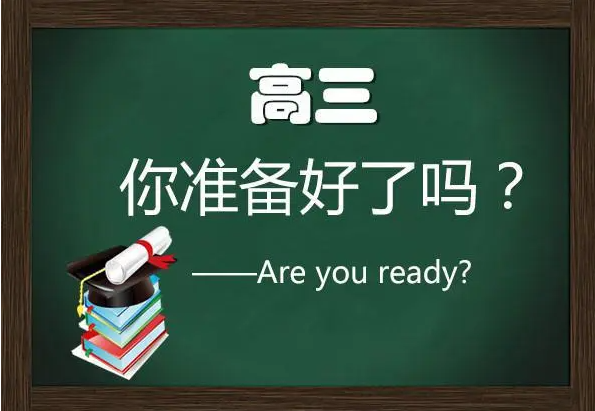﻿

## 高考数学知识点复习大全,一步一步走上高校大门!.由教育壹周刊整理的高考数学知识点复习大全,内容如下:

一、高考数学必考公式知识点

1.适用条件:[直线过焦点],必有ecosA=(x-1)/(x+1),其中A为直线与焦点所在轴夹角,是锐角。x为分离比,必须大于1。注上述公式适合一切圆锥曲线。如果焦点内分(指的是焦点在所截线段上),用该公式;如果外分(焦点在所截线段延长线上),右边为(x+1)/(x-1),其他不变。

2.函数的周期性问题(记忆三个):

(1)若f(x)=-f(x+k),则T=2k;

(2)若f(x)=m/(x+k)(m不为0),则T=2k;

(3)若f(x)=f(x+k)+f(x-k),则T=6k。注意点:a.周期函数,

周期必无限b.周期函数未必存在最小周期,如:常数函数。c.周期函数加周期函数未必是周期函数,如:y=sinxy=sin派x相加不是周期函数。

3.关于对称问题(无数人搞不懂的问题)总结如下:

(1)若在R上(下同)满足:f(a+x)=f(b-x)恒成立,对称轴为x=(a+b)/2

(2)函数y=f(a+x)与y=f(b-x)的图像关于x=(b-a)/2对称

(3)若f(a+x)+f(a-x)=2b,则f(x)图像关于(a,b)中心对称

高考数学知识点复习大纲

一、高考数学有函数、数列、三角函数、平面向量、不等式、立体几何等九章。

主要测试函数和导数,这是我们整个高中的核心部分。在这一部分中,我们关注两个方面:第一个函数的性质,包括函数的单调性和奇偶性;第二个是函数的答案,重点是二次函数和高次函数、分数函数及其一些分布问题,但分布重点还包括两个分析是二次方程的分布,这是第一部分。

二是平面向量和三角函数。

重点关注三个方面:一是减值,一是重点关注公式,重点关注五组基本公式。二是三角函数的图像和性质,重点是正弦函数和余弦函数的性质,三是正弦定理和余弦定理。难度相对较小。

第三、数列。

数列这个板块,重点考两个方面:一个通项;一是求和。

第四,空间向量和立体几何,重点关注两个方面:一是证明;一是计算。

第五、概率和统计。

这一部分主要属于数学应用问题的范畴。当然,我们应该掌握以下几个方面:一是可能的概率,二是事件,三是独立事件和独立重复事件的概率。

第六、押轴题。

考生在备考复习时,应注重不等式计算方法。虽然很难说,但我建议考生不要在整个试卷中留下空白。这是高考七大核心考点。高考数学知识点复习大全,教育壹周刊给大家整理的内容大致就是这些了,除了数学外,语文、英语等等,所占的比分也是很大的,到了最后的复习阶段,考生们要注意时间分配,还是不要把注意力全部放在一科上面喔。